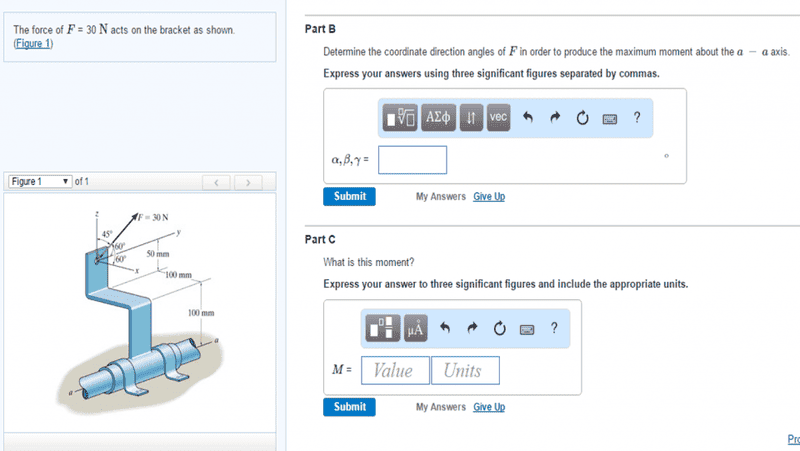# How to find the maximum moment of a force about an axis?

• marsupialofdeath

## Homework Statement

Calculating the moment of force F about the axis isn't hard; how do I determine how to produce the maximum moment?## Homework Equations

Maxis = ûaxis ⋅ (r×F)
F = 30N (This doesn't change at all during the problem)

## The Attempt at a Solution

I would guess that since the problem axis is parallel to the y-axis, you should minimize that component of the force (β=90°). I'm not totally sure on that point though. As for the other two axes, I have no idea.

Last edited:

I would guess that since the problem axis is parallel to the y-axis, you should minimize that component of the force
That is certainly true, but the diagram does not even suggest F having such a component. It only shows x and z components.
What is the magnitude of rxF in terms of the magnitudes of the vectors and the angle between them?

That is certainly true, but the diagram does not even suggest F having such a component. It only shows x and z components.
What is the magnitude of rxF in terms of the magnitudes of the vectors and the angle between them?
It's kind of hard to see in the diagram, but F is 60° from both the x and y axes.
Fx = F*cos(α) = 30*cos(60°) = 15 N
Fy = F*cos(β) = 30*cos(60°) = 15 N
Fz = F*cos(γ) = 30*cos(45°) ≈ 21.21 N
So, the vector for F should be (15i + 15j + 21.21k) N

And the vector for r is (-0.1i + 0.15k) m
So, r×F is (2.25i + 4.37j - 1.5k) N⋅m... That is the moment of force about the center of the given axis-- dot that with the unit vector j to determine that with the current angles, the moment about the axis is just 4.37 N⋅m. And we're trying to maximize that, so we need to maximize the j component of the r×F vector.

I'm not completely sure what you are asking, but I hope that clears things up a bit.

First, I withdraw my previous remark. On enlarging the diagram I see that it shows F as having a y component.
However, the question asks for the directions that would maximise the moment for the given magnitude. That surely means you should ignore the given angles for this part of the question.

That surely means you should ignore the given angles for this part of the question.
Yeah, the first part of the question asked for the moment about the axis, which is what those angles were used for. So we need to manipulate the force vector so that instead of the given angles, it's in the x-z plane. So can we set it up so that Fx = 30cos(α) and Fz = 30cos(γ) = 30sin(α)? From there maybe we can do r×F again and derive it and set that equal to zero so we can solve for α.

From there maybe we can do r×F again and derive it and set that equal to zero so we can solve for α.
That would work, but it is unnecessarily complicated.
Try to answer the question I asked at the end of post #2.